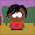## Friday, October 23, 2009

### How quantum is biology?

There was a really good discussion at the conference which clarified a few things for me.

Just how quantum are the effects (interference of excitons) that have been observed?
John Briggs asked how this was different from coupled harmonic oscillators?
Where does h-bar enter?
Shaul Mukamel pointed out that as long as one is looking in the one exciton sector the Heisenberg equations of motion just look like classical harmonic oscillators. hbar only enters as omega=frequency=E/hbar.

This is just like how the Bloch equations can be viewed as equations for a damped harmonic oscillator.
These questions about true quantum effects are reminiscent of the birth of quantum optics. People thought photons were necessary to explain spectroscopy. But actually this could all be explained in a semi-classical framework. It was only with experiments such as photon anti-bunching and squeezing that the truely quantum nature of light was established.

To see true quantum effects such as entanglement one will need to look in the two-exciton sector. Then a definitive signature of entanglement would be violation of Bell inequalities. Until such an experiment has been performed I see no justification for making claims that entanglement does exist in photosynthetic light harvesting systems.

Extra-ordinary claims require extra-ordinary evidence.

#### 1 comment:

1.Hi Ross,
It's true that the Redfield equations for a set of harmonic
oscillators are the same as those for excitations in a chromophoric
complex in the single excitation manifold. However, this seems like a
very weak objection to me. Firstly, we know very well that the
underlying physics is not described by a set of classical oscillators
(e.g. multiple exciton physics is experimentally accessible and
doesn't agree with the behavior of a set of classical oscillators). So
the objection that the 2D spectroscopy signals could have just as well come from a set of oscillators is kind of strange. Second, the reason first-order coherence is not sufficient to characterize the quantum properties of an EM field is that underlying physical model for the field is a set of oscillators. So in this case, to distinguish between classical and quantum oscillators, we need the g2 coherence at least. In contrast, once one accepts that the underlying model for excitation dynamics is based on *non-harmonic* electronic degrees of freedom then to conclude quantum properties, such as entanglement, from the density matrix in the single exciton manifold is fine.

~mohan